# Structural Analysis and Shape Descriptors¶

## moments¶

Calculates all of the moments up to the third order of a polygon or rasterized shape.

C++: Moments moments(InputArray array, bool binaryImage=false )
Python: cv2.moments(array[, binaryImage]) → retval
C: void cvMoments(const CvArr* arr, CvMoments* moments, int binary=0 )
Python: cv.Moments(arr, binary=0) → moments
Parameters: array – Raster image (single-channel, 8-bit or floating-point 2D array) or an array (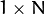or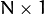) of 2D points (Point or Point2f ). binaryImage – If it is true, all non-zero image pixels are treated as 1’s. The parameter is used for images only. moments – Output moments.

The function computes moments, up to the 3rd order, of a vector shape or a rasterized shape. The results are returned in the structure Moments defined as:

class Moments
{
public:
Moments();
Moments(double m00, double m10, double m01, double m20, double m11,
double m02, double m30, double m21, double m12, double m03 );
Moments( const CvMoments& moments );
operator CvMoments() const;

// spatial moments
double  m00, m10, m01, m20, m11, m02, m30, m21, m12, m03;
// central moments
double  mu20, mu11, mu02, mu30, mu21, mu12, mu03;
// central normalized moments
double  nu20, nu11, nu02, nu30, nu21, nu12, nu03;
}


In case of a raster image, the spatial moments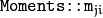are computed as: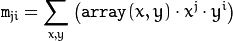The central momentsare computed as: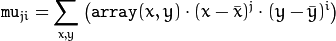where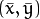is the mass center: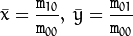The normalized central moments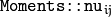are computed as: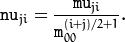Note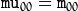,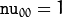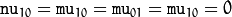, hence the values are not stored.

The moments of a contour are defined in the same way but computed using the Green’s formula (see http://en.wikipedia.org/wiki/Green_theorem). So, due to a limited raster resolution, the moments computed for a contour are slightly different from the moments computed for the same rasterized contour.

Note

Since the contour moments are computed using Green formula, you may get seemingly odd results for contours with self-intersections, e.g. a zero area (m00) for butterfly-shaped contours.

## HuMoments¶

Calculates seven Hu invariants.

C++: void HuMoments(const Moments& m, OutputArray hu)
C++: void HuMoments(const Moments& moments, double hu)
Python: cv2.HuMoments(m[, hu]) → hu
C: void cvGetHuMoments(CvMoments* moments, CvHuMoments* hu_moments)
Python: cv.GetHuMoments(moments) → hu
Parameters: moments – Input moments computed with moments() . hu – Output Hu invariants.

The function calculates seven Hu invariants (introduced in [Hu62]; see also http://en.wikipedia.org/wiki/Image_moment) defined as: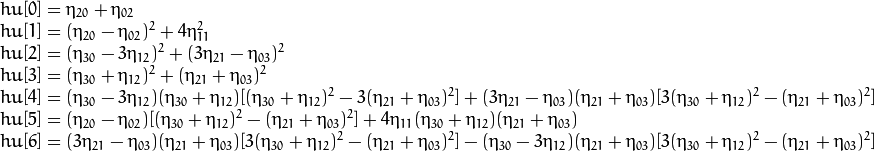where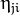stands for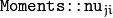.

These values are proved to be invariants to the image scale, rotation, and reflection except the seventh one, whose sign is changed by reflection. This invariance is proved with the assumption of infinite image resolution. In case of raster images, the computed Hu invariants for the original and transformed images are a bit different.

matchShapes()

## findContours¶

Finds contours in a binary image.

C++: void findContours(InputOutputArray image, OutputArrayOfArrays contours, OutputArray hierarchy, int mode, int method, Point offset=Point())
C++: void findContours(InputOutputArray image, OutputArrayOfArrays contours, int mode, int method, Point offset=Point())
Python: cv2.findContours(image, mode, method[, contours[, hierarchy[, offset]]]) → contours, hierarchy
C: int cvFindContours(CvArr* image, CvMemStorage* storage, CvSeq** first_contour, int header_size=sizeof(CvContour), int mode=CV_RETR_LIST, int method=CV_CHAIN_APPROX_SIMPLE, CvPoint offset=cvPoint(0,0) )
Python: cv.FindContours(image, storage, mode=CV_RETR_LIST, method=CV_CHAIN_APPROX_SIMPLE, offset=(0, 0)) → contours
Parameters: image – Source, an 8-bit single-channel image. Non-zero pixels are treated as 1’s. Zero pixels remain 0’s, so the image is treated as binary . You can use compare() , inRange() , threshold() , adaptiveThreshold() , Canny() , and others to create a binary image out of a grayscale or color one. The function modifies the image while extracting the contours. contours – Detected contours. Each contour is stored as a vector of points. hierarchy – Optional output vector containing information about the image topology. It has as many elements as the number of contours. For each contour contours[i] , the elements hierarchy[i] , hiearchy[i] , hiearchy[i] , and hiearchy[i] are set to 0-based indices in contours of the next and previous contours at the same hierarchical level: the first child contour and the parent contour, respectively. If for a contour i there are no next, previous, parent, or nested contours, the corresponding elements of hierarchy[i] will be negative. mode – Contour retrieval mode (if you use Python see also a note below). CV_RETR_EXTERNAL retrieves only the extreme outer contours. It sets hierarchy[i]=hierarchy[i]=-1 for all the contours. CV_RETR_LIST retrieves all of the contours without establishing any hierarchical relationships. CV_RETR_CCOMP retrieves all of the contours and organizes them into a two-level hierarchy. At the top level, there are external boundaries of the components. At the second level, there are boundaries of the holes. If there is another contour inside a hole of a connected component, it is still put at the top level. CV_RETR_TREE retrieves all of the contours and reconstructs a full hierarchy of nested contours. This full hierarchy is built and shown in the OpenCV contours.c demo. method – Contour approximation method (if you use Python see also a note below). CV_CHAIN_APPROX_NONE stores absolutely all the contour points. That is, any 2 subsequent points (x1,y1) and (x2,y2) of the contour will be either horizontal, vertical or diagonal neighbors, that is, max(abs(x1-x2),abs(y2-y1))==1. CV_CHAIN_APPROX_SIMPLE compresses horizontal, vertical, and diagonal segments and leaves only their end points. For example, an up-right rectangular contour is encoded with 4 points. CV_CHAIN_APPROX_TC89_L1,CV_CHAIN_APPROX_TC89_KCOS applies one of the flavors of the Teh-Chin chain approximation algorithm. See [TehChin89] for details. offset – Optional offset by which every contour point is shifted. This is useful if the contours are extracted from the image ROI and then they should be analyzed in the whole image context.

The function retrieves contours from the binary image using the algorithm [Suzuki85]. The contours are a useful tool for shape analysis and object detection and recognition. See squares.c in the OpenCV sample directory.

Note

Source image is modified by this function.

Note

If you use the new Python interface then the CV_ prefix has to be omitted in contour retrieval mode and contour approximation method parameters (for example, use cv2.RETR_LIST and cv2.CHAIN_APPROX_NONE parameters). If you use the old Python interface then these parameters have the CV_ prefix (for example, use cv.CV_RETR_LIST and cv.CV_CHAIN_APPROX_NONE).

## approxPolyDP¶

Approximates a polygonal curve(s) with the specified precision.

C++: void approxPolyDP(InputArray curve, OutputArray approxCurve, double epsilon, bool closed)
Python: cv2.approxPolyDP(curve, epsilon, closed[, approxCurve]) → approxCurve
C: CvSeq* cvApproxPoly(const void* src_seq, int header_size, CvMemStorage* storage, int method, double eps, int recursive=0 )
Parameters: curve – Input vector of a 2D point stored in: std::vector or Mat (C++ interface) Nx2 numpy array (Python interface) CvSeq or  CvMat (C interface) approxCurve – Result of the approximation. The type should match the type of the input curve. In case of C interface the approximated curve is stored in the memory storage and pointer to it is returned. epsilon – Parameter specifying the approximation accuracy. This is the maximum distance between the original curve and its approximation. closed – If true, the approximated curve is closed (its first and last vertices are connected). Otherwise, it is not closed. header_size – Header size of the approximated curve. Normally, sizeof(CvContour) is used. storage – Memory storage where the approximated curve is stored. method – Contour approximation algorithm. Only CV_POLY_APPROX_DP is supported. recursive – Recursion flag. If it is non-zero and curve is CvSeq*, the function cvApproxPoly approximates all the contours accessible from curve by h_next and v_next links.

The functions approxPolyDP approximate a curve or a polygon with another curve/polygon with less vertices so that the distance between them is less or equal to the specified precision. It uses the Douglas-Peucker algorithm http://en.wikipedia.org/wiki/Ramer-Douglas-Peucker_algorithm

See http://code.opencv.org/svn/opencv/trunk/opencv/samples/cpp/contours.cpp for the function usage model.

## ApproxChains¶

Approximates Freeman chain(s) with a polygonal curve.

C: CvSeq* cvApproxChains(CvSeq* src_seq, CvMemStorage* storage, int method=CV_CHAIN_APPROX_SIMPLE, double parameter=0, int minimal_perimeter=0, int recursive=0 )
Python: cv.ApproxChains(src_seq, storage, method=CV_CHAIN_APPROX_SIMPLE, parameter=0, minimal_perimeter=0, recursive=0) → contours
Parameters: src_seq – Pointer to the approximated Freeman chain that can refer to other chains. storage – Storage location for the resulting polylines. method – Approximation method (see the description of the function FindContours() ). parameter – Method parameter (not used now). minimal_perimeter – Approximates only those contours whose perimeters are not less than minimal_perimeter . Other chains are removed from the resulting structure. recursive – Recursion flag. If it is non-zero, the function approximates all chains that can be obtained from chain by using the h_next or v_next links. Otherwise, the single input chain is approximated.

This is a standalone contour approximation routine, not represented in the new interface. When FindContours() retrieves contours as Freeman chains, it calls the function to get approximated contours, represented as polygons.

## arcLength¶

Calculates a contour perimeter or a curve length.

C++: double arcLength(InputArray curve, bool closed)
Python: cv2.arcLength(curve, closed) → retval
C: double cvArcLength(const void* curve, CvSlice slice=CV_WHOLE_SEQ, int is_closed=-1 )
Python: cv.ArcLength(curve, slice=CV_WHOLE_SEQ, isClosed=-1) → float
Parameters: curve – Input vector of 2D points, stored in std::vector or Mat. closed – Flag indicating whether the curve is closed or not.

The function computes a curve length or a closed contour perimeter.

## boundingRect¶

Calculates the up-right bounding rectangle of a point set.

C++: Rect boundingRect(InputArray points)
Python: cv2.boundingRect(points) → retval
C: CvRect cvBoundingRect(CvArr* points, int update=0 )
Python: cv.BoundingRect(points, update=0) → CvRect
Parameters: points – Input 2D point set, stored in std::vector or Mat.

The function calculates and returns the minimal up-right bounding rectangle for the specified point set.

## contourArea¶

Calculates a contour area.

C++: double contourArea(InputArray contour, bool oriented=false )
Python: cv2.contourArea(contour[, oriented]) → retval
C: double cvContourArea(const CvArr* contour, CvSlice slice=CV_WHOLE_SEQ, int oriented=0 )
Python: cv.ContourArea(contour, slice=CV_WHOLE_SEQ) → float
Parameters: contour – Input vector of 2D points (contour vertices), stored in std::vector or Mat. oriented – Oriented area flag. If it is true, the function returns a signed area value, depending on the contour orientation (clockwise or counter-clockwise). Using this feature you can determine orientation of a contour by taking the sign of an area. By default, the parameter is false, which means that the absolute value is returned.

The function computes a contour area. Similarly to moments() , the area is computed using the Green formula. Thus, the returned area and the number of non-zero pixels, if you draw the contour using drawContours() or fillPoly() , can be different. Also, the function will most certainly give a wrong results for contours with self-intersections.

Example:

vector<Point> contour;
contour.push_back(Point2f(0, 0));
contour.push_back(Point2f(10, 0));
contour.push_back(Point2f(10, 10));
contour.push_back(Point2f(5, 4));

double area0 = contourArea(contour);
vector<Point> approx;
approxPolyDP(contour, approx, 5, true);
double area1 = contourArea(approx);

cout << "area0 =" << area0 << endl <<
"area1 =" << area1 << endl <<
"approx poly vertices" << approx.size() << endl;


## convexHull¶

Finds the convex hull of a point set.

C++: void convexHull(InputArray points, OutputArray hull, bool clockwise=false, bool returnPoints=true )
Python: cv2.convexHull(points[, hull[, clockwise[, returnPoints]]]) → hull
C: CvSeq* cvConvexHull2(const CvArr* input, void* hull_storage=NULL, int orientation=CV_CLOCKWISE, int return_points=0 )
Python: cv.ConvexHull2(points, storage, orientation=CV_CLOCKWISE, return_points=0) → convexHull
Parameters: points – Input 2D point set, stored in std::vector or Mat. hull – Output convex hull. It is either an integer vector of indices or vector of points. In the first case, the hull elements are 0-based indices of the convex hull points in the original array (since the set of convex hull points is a subset of the original point set). In the second case, hull elements are the convex hull points themselves. hull_storage – Output memory storage in the old API (cvConvexHull2 returns a sequence containing the convex hull points or their indices). clockwise – Orientation flag. If it is true, the output convex hull is oriented clockwise. Otherwise, it is oriented counter-clockwise. The usual screen coordinate system is assumed so that the origin is at the top-left corner, x axis is oriented to the right, and y axis is oriented downwards. orientation – Convex hull orientation parameter in the old API, CV_CLOCKWISE or CV_COUNTERCLOCKWISE. returnPoints – Operation flag. In case of a matrix, when the flag is true, the function returns convex hull points. Otherwise, it returns indices of the convex hull points. When the output array is std::vector, the flag is ignored, and the output depends on the type of the vector: std::vector implies returnPoints=true, std::vector implies returnPoints=false.

The functions find the convex hull of a 2D point set using the Sklansky’s algorithm [Sklansky82] that has O(N logN) complexity in the current implementation. See the OpenCV sample convexhull.cpp that demonstrates the usage of different function variants.

## convexityDefects¶

Finds the convexity defects of a contour.

C++: void convexityDefects(InputArray contour, InputArray convexhull, OutputArray convexityDefects)
Python: cv2.convexityDefects(contour, convexhull[, convexityDefects]) → convexityDefects
C: CvSeq* cvConvexityDefects(const CvArr* contour, const CvArr* convexhull, CvMemStorage* storage=NULL )
Python: cv.ConvexityDefects(contour, convexhull, storage) → convexityDefects
Parameters: contour – Input contour. convexhull – Convex hull obtained using convexHull() that should contain indices of the contour points that make the hull. convexityDefects – The output vector of convexity defects. In C++ and the new Python/Java interface each convexity defect is represented as 4-element integer vector (a.k.a. cv::Vec4i): (start_index, end_index, farthest_pt_index, fixpt_depth), where indices are 0-based indices in the original contour of the convexity defect beginning, end and the farthest point, and fixpt_depth is fixed-point approximation (with 8 fractional bits) of the distance between the farthest contour point and the hull. That is, to get the floating-point value of the depth will be fixpt_depth/256.0. In C interface convexity defect is represented by CvConvexityDefect structure - see below. storage – Container for the output sequence of convexity defects. If it is NULL, the contour or hull (in that order) storage is used.

The function finds all convexity defects of the input contour and returns a sequence of the CvConvexityDefect structures, where CvConvexityDetect is defined as:

struct CvConvexityDefect
{
CvPoint* start; // point of the contour where the defect begins
CvPoint* end; // point of the contour where the defect ends
CvPoint* depth_point; // the farthest from the convex hull point within the defect
float depth; // distance between the farthest point and the convex hull
};


The figure below displays convexity defects of a hand contour: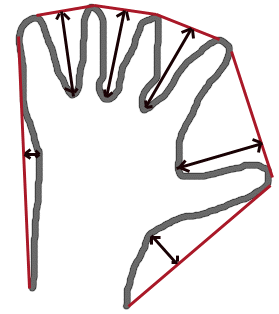## fitEllipse¶

Fits an ellipse around a set of 2D points.

C++: RotatedRect fitEllipse(InputArray points)
Python: cv2.fitEllipse(points) → retval
C: CvBox2D cvFitEllipse2(const CvArr* points)
Python: cv.FitEllipse2(points) → Box2D
Parameters: points – Input 2D point set, stored in: std::vector<> or Mat (C++ interface) CvSeq* or CvMat* (C interface) Nx2 numpy array (Python interface)

The function calculates the ellipse that fits (in a least-squares sense) a set of 2D points best of all. It returns the rotated rectangle in which the ellipse is inscribed. The algorithm [Fitzgibbon95] is used.

## fitLine¶

Fits a line to a 2D or 3D point set.

C++: void fitLine(InputArray points, OutputArray line, int distType, double param, double reps, double aeps)
Python: cv2.fitLine(points, distType, param, reps, aeps[, line]) → line
C: void cvFitLine(const CvArr* points, int dist_type, double param, double reps, double aeps, float* line)
Python: cv.FitLine(points, dist_type, param, reps, aeps) → line
Parameters: points – Input vector of 2D or 3D points, stored in std::vector<> or Mat. line – Output line parameters. In case of 2D fitting, it should be a vector of 4 elements (like Vec4f) - (vx, vy, x0, y0), where (vx, vy) is a normalized vector collinear to the line and (x0, y0) is a point on the line. In case of 3D fitting, it should be a vector of 6 elements (like Vec6f) - (vx, vy, vz, x0, y0, z0), where (vx, vy, vz) is a normalized vector collinear to the line and (x0, y0, z0) is a point on the line. distType – Distance used by the M-estimator (see the discussion below). param – Numerical parameter ( C ) for some types of distances. If it is 0, an optimal value is chosen. reps – Sufficient accuracy for the radius (distance between the coordinate origin and the line). aeps – Sufficient accuracy for the angle. 0.01 would be a good default value for reps and aeps.

The function fitLine fits a line to a 2D or 3D point set by minimizing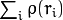where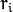is a distance between the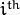point, the line and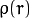is a distance function, one of the following:

• distType=CV_DIST_L2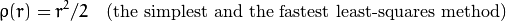• distType=CV_DIST_L1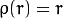• distType=CV_DIST_L12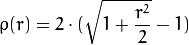• distType=CV_DIST_FAIR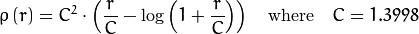• distType=CV_DIST_WELSCH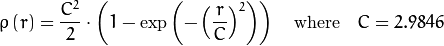• distType=CV_DIST_HUBER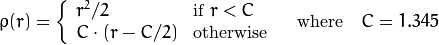The algorithm is based on the M-estimator ( http://en.wikipedia.org/wiki/M-estimator ) technique that iteratively fits the line using the weighted least-squares algorithm. After each iteration the weights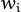are adjusted to be inversely proportional to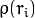.

## isContourConvex¶

Tests a contour convexity.

C++: bool isContourConvex(InputArray contour)
Python: cv2.isContourConvex(contour) → retval
C: int cvCheckContourConvexity(const CvArr* contour)
Python: cv.CheckContourConvexity(contour) → int
Parameters: contour – Input vector of 2D points, stored in: std::vector<> or Mat (C++ interface) CvSeq* or CvMat* (C interface) Nx2 numpy array (Python interface)

The function tests whether the input contour is convex or not. The contour must be simple, that is, without self-intersections. Otherwise, the function output is undefined.

## minAreaRect¶

Finds a rotated rectangle of the minimum area enclosing the input 2D point set.

C++: RotatedRect minAreaRect(InputArray points)
Python: cv2.minAreaRect(points) → retval
C: CvBox2D cvMinAreaRect2(const CvArr* points, CvMemStorage* storage=NULL )
Python: cv.MinAreaRect2(points, storage=None) → Box2D
Parameters: points – Input vector of 2D points, stored in: std::vector<> or Mat (C++ interface) CvSeq* or CvMat* (C interface) Nx2 numpy array (Python interface)

The function calculates and returns the minimum-area bounding rectangle (possibly rotated) for a specified point set. See the OpenCV sample minarea.cpp .

## minEnclosingCircle¶

Finds a circle of the minimum area enclosing a 2D point set.

C++: void minEnclosingCircle(InputArray points, Point2f& center, float& radius)
Python: cv2.minEnclosingCircle(points) → center, radius
C: int cvMinEnclosingCircle(const CvArr* points, CvPoint2D32f* center, float* radius)
Python: cv.MinEnclosingCircle(points)-> (int, center, radius)
Parameters: points – Input vector of 2D points, stored in: std::vector<> or Mat (C++ interface) CvSeq* or CvMat* (C interface) Nx2 numpy array (Python interface) center – Output center of the circle. radius – Output radius of the circle.

The function finds the minimal enclosing circle of a 2D point set using an iterative algorithm. See the OpenCV sample minarea.cpp .

## matchShapes¶

Compares two shapes.

C++: double matchShapes(InputArray contour1, InputArray contour2, int method, double parameter)
Python: cv2.matchShapes(contour1, contour2, method, parameter) → retval
C: double cvMatchShapes(const void* object1, const void* object2, int method, double parameter=0 )
Python: cv.MatchShapes(object1, object2, method, parameter=0) → float
Parameters: object1 – First contour or grayscale image. object2 – Second contour or grayscale image. method – Comparison method: CV_CONTOURS_MATCH_I1 , CV_CONTOURS_MATCH_I2 or CV_CONTOURS_MATCH_I3 (see the details below). parameter – Method-specific parameter (not supported now).

The function compares two shapes. All three implemented methods use the Hu invariants (see HuMoments() ) as follows (denotes object1,:math:B denotes object2 ):

• method=CV_CONTOURS_MATCH_I1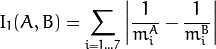• method=CV_CONTOURS_MATCH_I2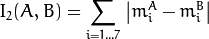• method=CV_CONTOURS_MATCH_I3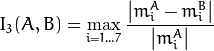where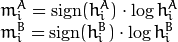and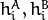are the Hu moments ofand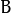, respectively.

## pointPolygonTest¶

Performs a point-in-contour test.

C++: double pointPolygonTest(InputArray contour, Point2f pt, bool measureDist)
Python: cv2.pointPolygonTest(contour, pt, measureDist) → retval
C: double cvPointPolygonTest(const CvArr* contour, CvPoint2D32f pt, int measure_dist)
Python: cv.PointPolygonTest(contour, pt, measure_dist) → float
Parameters: contour – Input contour. pt – Point tested against the contour. measureDist – If true, the function estimates the signed distance from the point to the nearest contour edge. Otherwise, the function only checks if the point is inside a contour or not.

The function determines whether the point is inside a contour, outside, or lies on an edge (or coincides with a vertex). It returns positive (inside), negative (outside), or zero (on an edge) value, correspondingly. When measureDist=false , the return value is +1, -1, and 0, respectively. Otherwise, the return value is a signed distance between the point and the nearest contour edge.

See below a sample output of the function where each image pixel is tested against the contour.[Fitzgibbon95] Andrew W. Fitzgibbon, R.B.Fisher. A Buyer’s Guide to Conic Fitting. Proc.5th British Machine Vision Conference, Birmingham, pp. 513-522, 1995.
 [Hu62] Hu. Visual Pattern Recognition by Moment Invariants, IRE Transactions on Information Theory, 8:2, pp. 179-187, 1962.
 [Sklansky82] Sklansky, J., Finding the Convex Hull of a Simple Polygon. PRL 1 \$number, pp 79-83 (1982)
 [Suzuki85] Suzuki, S. and Abe, K., Topological Structural Analysis of Digitized Binary Images by Border Following. CVGIP 30 1, pp 32-46 (1985)
 [TehChin89] Teh, C.H. and Chin, R.T., On the Detection of Dominant Points on Digital Curve. PAMI 11 8, pp 859-872 (1989)